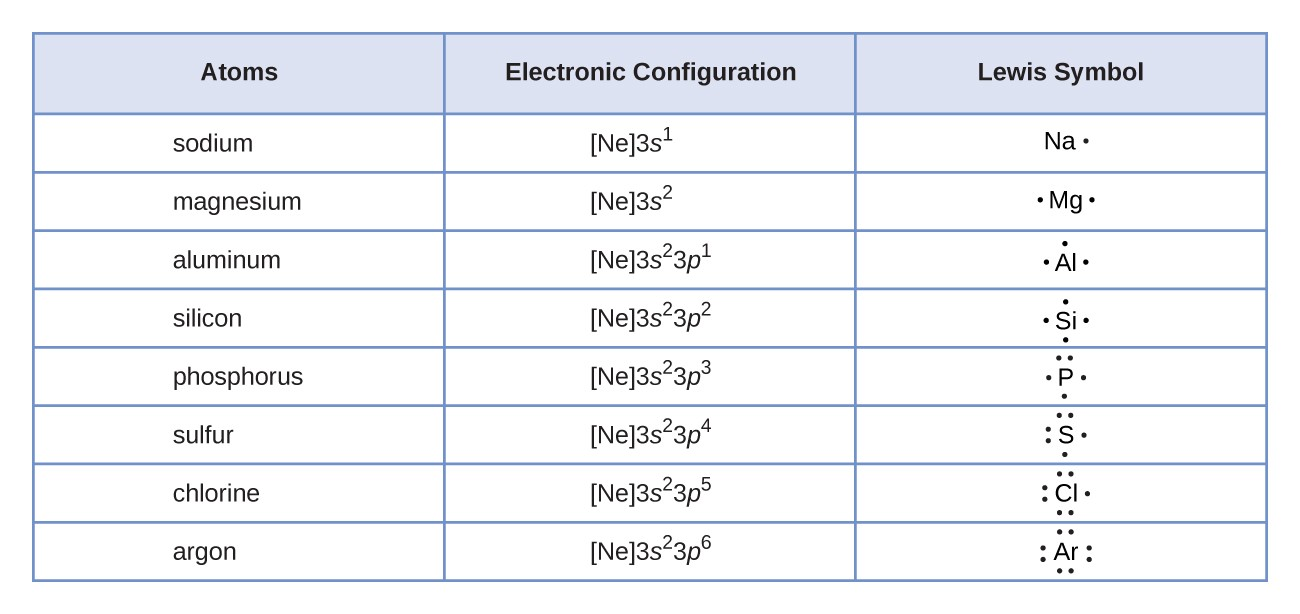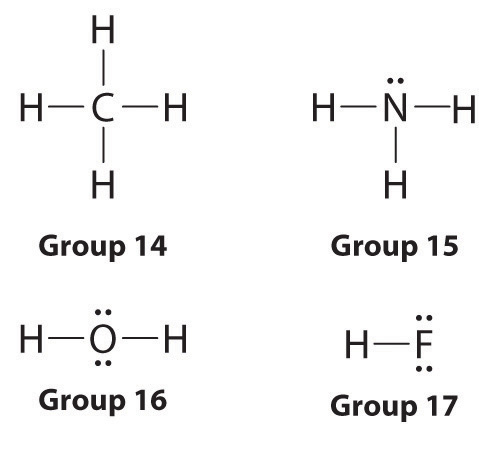# Electron Dot Structure Study Guide

Introduction

You probably already know what an electron is; they’re the negatively charged particles that orbit the nucleus. But do you know what electron configuration is?

There is a standard notation that is followed to represent the number of electrons distributed in a particular element at an atomic orbital, which helps determine the particular sequence of electrons contained in the atomic subshell. This electron distribution configuration is done either through the electron dot pictures or Lewis structures.

### What is an electron dot structure?

An electron dot structure, also known as a Lewis dot formula, is a representation of the electrons found in the valence shell of an atom. Drawing electron dot diagrams is possible if you know the molecular formula of the compound. They help define the nature of the bond and the position of atoms which are connected in molecules. This structure showing electron distribution is in honor of the American chemist Gilbert Newton Lewis.

Lewis structures help you understand the chemical bonding between atoms in a molecule. They also help determine the lone pairs present in an atom. Lewis established a base as an electron pair donor and an acid as an electron pair acceptor. Lewis dot structure is often thought of as ‘electron bookkeeping.’

### How to draw an electron dot diagram

For a chemistry student attempting to understand electron configurations for the first time, Lewis structures might be a little confusing. But once you understand the concept, it’s a pretty straightforward method of learning. Let’s go over the steps of how to make one.Source

Step 1: Figure out the total number of valence electrons

Add the number of valence electrons present in the molecule.

Step 2: Find how to satisfy the outermost shell of the atom

The number of electrons in the valence shell of an atom largely determines its reactivity and tendency to form bonds with other atoms. Atoms tend to prefer to have 8 electrons in this shell, a phenomenon labeled as the octet rule.

Step 3: Figure out the number of bonds

A covalent bond is formed when two atoms in a molecule share an electron. Determine the number of electrons you have and the number of electrons you need, then subtract the former from the latter. The bond formed requires two electrons; therefore, the number of bonds is exactly half the number of electrons needed.

Step 4: Select a central atom

The least electronegative atom is the central atom of a molecule. You can determine this value using the periodic table.

Step 5: Draw the structure by placing electrons around the center

After selecting the central atom, join the other atoms in the molecule to it using a straight line, which represents a bond. The central atom can have up to four other atoms connected to it. Follow the octet rule and finish filling the outer shell. If there aren’t ample electrons to complete the octet, then through trial and error, you need to figure out the right skeletal structure. If there are any bonds left, then create double bonds with lone pairs on outside atoms.Source

## CONCLUSION:

• Electron dot structures represent the nature of a bond between two atoms and their positions in a molecule.
• Lewis dot structures are often thought of as ‘electron bookkeeping’.

### FAQs:

1. What are electron dot diagrams?

The electron dot diagram is a representation of the valence electrons of an atom that uses dots around the symbol of the element.

2. How do you draw an electron dot diagram for an element?

• Find the total number of valence electrons
• Find the number of electrons that would satisfy the outermost shell
• Determine the number of bonds
• Choose a central atom
• Draw skeletal structure and place electrons

We hope you enjoyed studying this lesson and learned something cool about How To Draw Electron Dot Structures! Join our Discord community to get any questions you may have answered and to engage with other students just like you! Don’t forget to download our app to experience our fun VR classrooms – we promise it makes studying much more fun! 😎

## SOURCES:

1. Lewis Dot Structure: https://byjus.com/chemistry/lewis-dot-structures/. Accessed 28 Feb 2022.
2. How to Draw a Lewis Structure: https://www.thoughtco.com/how-to-draw-a-lewis-structure-603983. Accessed 28 Feb 2022.
3. Electron Dot Diagram: https://www.ck12.org/c/chemistry/electron-dot-diagrams/?difficulty=all&by=all&modalityGroupName=video&filterReferrer=search. Accessed 28 Feb 2022.
]]>